# How to calculate days left in a month or year in Excel?

At times, you may want to know the working days left in a month or in a year. So, if you want to calculate the remaining days left in a month or to calculate the remaining days left in a year you can follow the below article which is easy and quick to calculate the days left in a month or in a year in an Excel sheet.

Follow the steps given below to calculate the days or working days left in a month.

## Step 1

Open an Excel sheet and enter the date for which you want to calculate the remaining days or working days left in that specific month as shown in the below screenshot for your reference.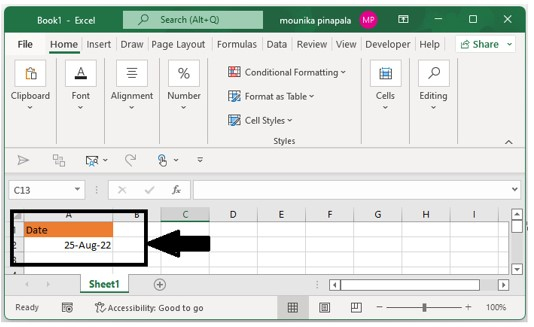## Step 2

Now you need to enter the below given formula and press enter key to calculate the days remaining or left in a specific month. Kindly find the below screenshot for your reference.

=EOMONTH(A2,0)-A2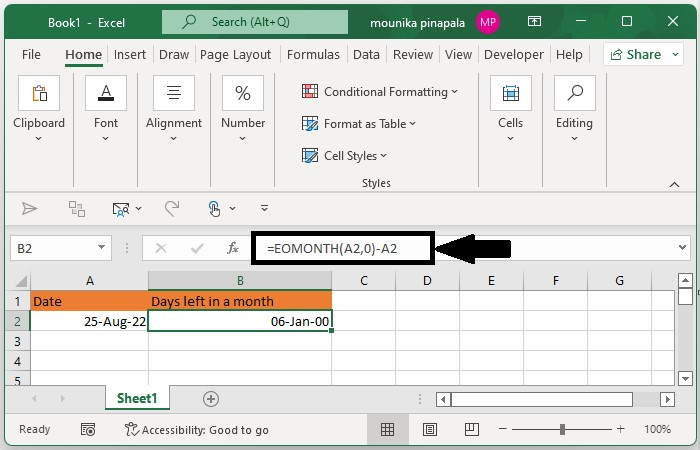## Step 3

If you see in the above screenshot days left in a month have been captured as a date. You need to change the format to general. To do this select the cell, and then you need to right click to choose format cells from the format cell dialog box and then click on general from the left category under the number tab as shown in the below screenshot for your reference.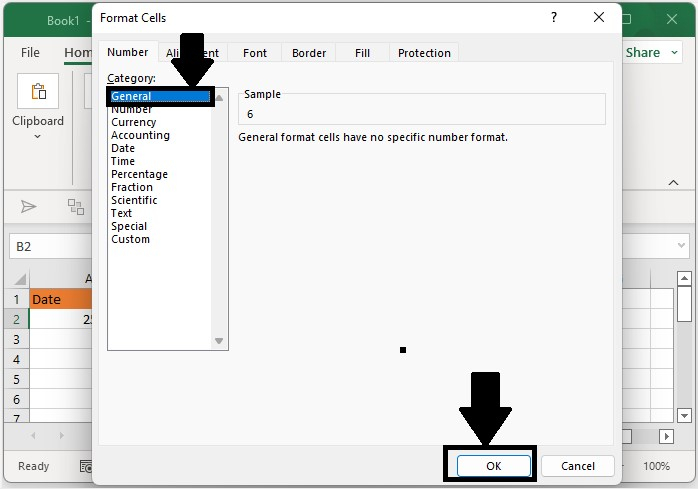## Step 4

Now you need to click on ok to see the remaining number of days left in a specific month given as shown in the below screenshot for your reference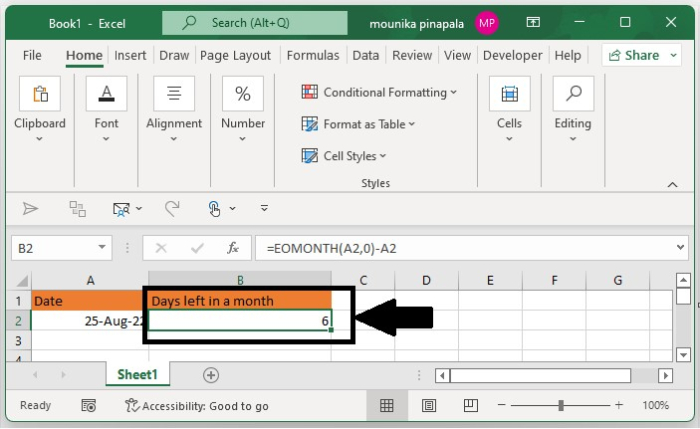Note − If you want to calculate only the working days left in a month then you need to use the formula =NETWORKDAY(A2,EOMONTH(A2,)) and then press enter key.

If you want to calculate the days left in a year for a given specific date, you need to use the below given formula. Enter the formula given below into the blank cell and then press enter key as shown in the below screenshot for your reference.

=DATE(YEAR(A2),12,31)-A2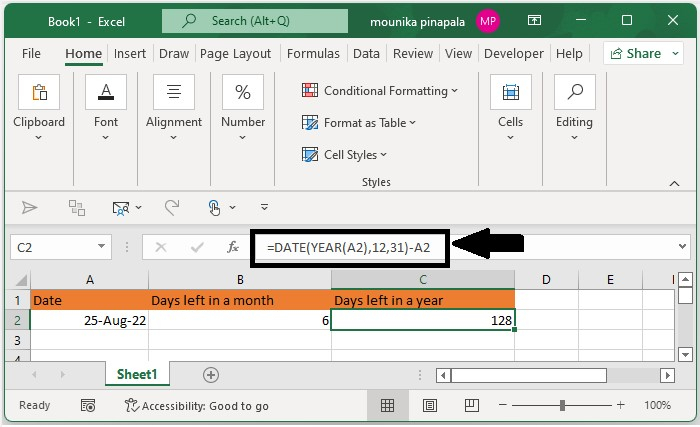## Conclusion

In this tutorial, we used a simple example to demonstrate how you can calculate the days left in a month or year in Excel.

Updated on: 03-Feb-2023

3K+ Views